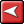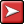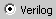## (TD) 6.7 Entering Direct HDL Code for Simulated Signals## (TD) 6.7 Entering Direct HDL Code for Simulated SignalsFor simplicity, the counter output COUNT is modeled using a simple block of behavioral HDL Code instead of using Boolean equations. It would take a large number of Boolean equations to model the counter and the equations would be difficult to modify if the counter operation had to be changed. For this tutorial we will create a 4-bit counter to test our system. This counter could be easily modified later to make it 12-bit (to acquire 4K worth of data). To enter direct HDL code for the COUNT signal:

1. Create a signal called COUNT.

2. In the Signal Properties dialog, set the Radix to hex, its MSB to 3, and check the Simulate radio button.

3. Press the Verilog radio buttonto switch from the Equation view to the HDL Code view/editor.

4. Enter the Verilog code below in the HDL Code editor of the Signal Properties dialog (comments begin with // and can be skipped during code entry). You can copy and paste the text into WaveFormer instead of typing it (Select and copy to clipboard the source code below, then click into the HDL Code window in WaveFormer and press <Ctrl>-V to paste the text):

reg [3:0] COUNTER;    //declare a 4-bit register called COUNTER

always @(negedge CLK0)        //on each falling edge of CLK0

begin

if (ENABLE)

COUNTER = COUNTER + 1;  // count while ENABLE is high

else

COUNTER = 0;    // synchronous reset if ENABLE is low

end

assign COUNT = COUNTER;   //drive wire COUNT with reg COUNTER value

5. Click the Simulate Once button to simulate the COUNT signal.

Note: All signals in WaveFormer are modeled as wires, so the assign is required at the end of the HDL code block to drive the COUNT wire with the value of COUNTER (which must be a register in order to remember its value).

To increase the size of the counter to acquire 4K data values (do not do this now), we could change the MSB of COUNT to 11 and change the declaration of COUNTER in the HDL code to:

reg [11:0] COUNTER;   //example only, don't do in this tutorial

[****]